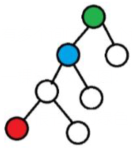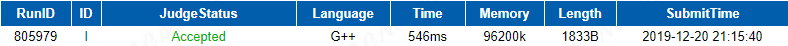## 题目描述

Time Limit: 12000 MS Memory Limit: 131072 K

DescriptionInput

Output

Sample Input
7 1
1 2
1 3
2 4
2 5
4 6
4 7
4
6 3
1 2
7 2
4 1

Sample Output
1
0
2
2

## AC代码

#include <bits/stdc++.h>
using namespace std;
const int N=1e6+10;
//f[i][j]表示i的2^j祖先，也就是i向上跳2^j步的点。dep表示深度。
struct node
{
int to,next;
}e[N<<1];
void add(int x,int y)
{
e[cnt].to=y;
}
void dfs(int u,int father)//预处理得到f数组，u表示当前搜索到的点，father表示u的父节点
{
dep[u]=dep[father]+1;//u的父节点深度+1就是u的深度
f[u]=father;//u向上跳2^0步(1步)，为father点
for(int i=1;i<=20;i++)//u与其祖先的距离最大不超过2^20
f[u][i]=f[f[u][i-1]][i-1];//dp的思想：u向上跳2^i步相当于u向上跳2^(i-1)步，再向上跳2^(i-1)步
{
int v=e[i].to;
if(v!=father)dfs(v,u);//如果当前遍历的点v不是father点，则可以向下继续搜索
}
}
int get_fa(int x,int k)
{
int t=dep[x]-k;
for(int i=20;i>=0;i--)//倍增向上跳跃，求祖先
if(dep[f[x][i]]>t)x=f[x][i];
return f[x];
}
int main()
{
ios::sync_with_stdio(false);
while(cin>>n>>s)
{
for(int i=1;i<=n-1;i++)
{
cin>>x>>y;
}
memset(f,0,sizeof(f));
dfs(s,0);//从起点s开始搜索，设起点的父节点为0
cin>>m;
while(m--)
{
cin>>x>>k;
printf("%d\n",get_fa(x,k));
}
}
return 0;
}


## 运行结果：